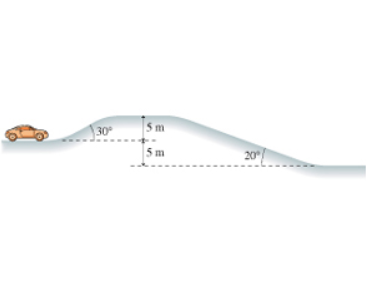# Problem: A 1400 kg car is approaching the hill shown in the figure at 13.0m/s when it suddenly runs out of gas. (Figure 1)What is the car's speed after coasting down the other side? in m/s

###### FREE Expert Solution

Kinetic energy:

$\overline{){\mathbf{K}}{\mathbf{E}}{\mathbf{=}}\frac{\mathbf{1}}{\mathbf{2}}{\mathbf{m}}{{\mathbf{v}}}^{{\mathbf{2}}}}$

Potential energy:

$\overline{){\mathbf{U}}{\mathbf{=}}{\mathbf{m}}{\mathbf{g}}{\mathbf{h}}}$

Conservation of energy:

KE0 + U0 = KEf + Uf

Uf = 0 (because hf = 0)

• m = 1400 kg
• v0 = 13.0m/s
• h0 = 5 m
• g = 9.8 m/s2

(1/2)mvf2 = (1/2)mv02 + mgh

94% (498 ratings)###### Problem Details

A 1400 kg car is approaching the hill shown in the figure at 13.0m/s when it suddenly runs out of gas. (Figure 1)

What is the car's speed after coasting down the other side? in m/sFrequently Asked Questions

What scientific concept do you need to know in order to solve this problem?

Our tutors have indicated that to solve this problem you will need to apply the Conservative Forces & Inclined Planes concept. You can view video lessons to learn Conservative Forces & Inclined Planes. Or if you need more Conservative Forces & Inclined Planes practice, you can also practice Conservative Forces & Inclined Planes practice problems.

What professor is this problem relevant for?

Based on our data, we think this problem is relevant for Professor Bonde's class at TCU.